Journal of Vibroengineering

Published: 15 November 2019

# Dynamics research of a flywheel shafting with PMB and a single point flexible support

Changliang Tang1
Bokang Su2
Xiaobo Liu3
1, 2, 3Key Laboratory of Modern Measurement and Control Technology, Ministry of Education, Beijing Information Science and Technology University, Beijing, 100192, China
Corresponding Author:
Changliang Tang
Views 163

#### Abstract

Flywheel energy storage system (FESS) is new advanced machinery which is intended for electric power storage and release. A FESS, which is suitable for the small flywheel, was developed with a permanent magnet bearing (PMB) and a single point flexible support, and its friction loss is very low. By means of the Lagrange theory, a dynamic model was established. The Campbell diagram, mode shapes, modal damping ratios and critical speeds were designed after the flywheel data at different operating speeds was obtained by numerical simulation. Based on the excitation test and single freedom vibration theory, the stiffness and damping coefficient of the upper and lower damper was measured. The influences of damper dynamic parameters on modes of flywheel rotor bearing system were discussed in detail. The comparison between the calculated unbalance response and the experimental response indicates that the dynamic model is appropriate. The results showed that the lower damper absorbed vibration energy of the flywheel rotor, which increased vibration of the damping body. And the bigger damping coefficient had better vibration absorption effect. The developed FESS is simple, stable and efficient in structure.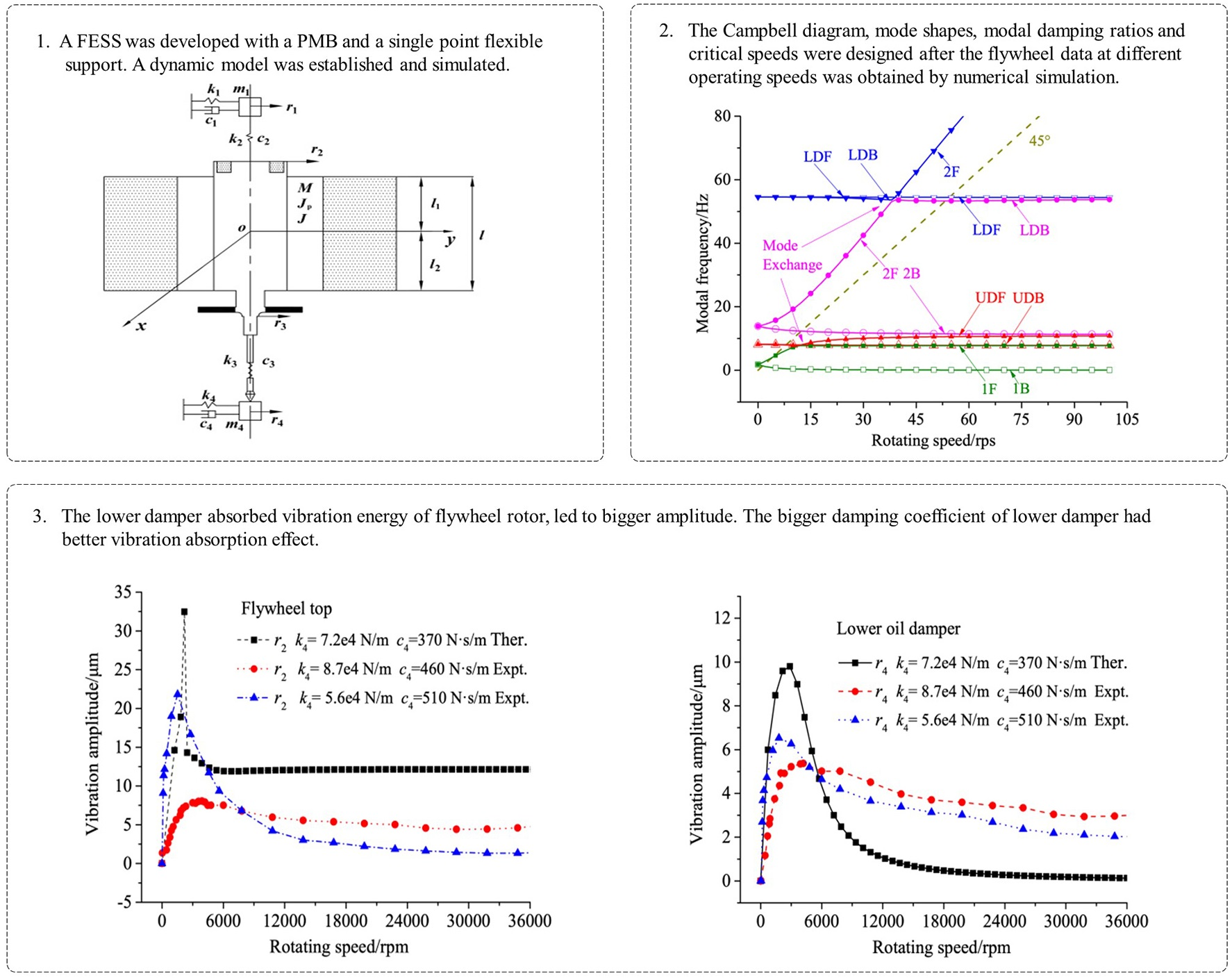#### Highlights

• A FESS was developed with a permanent magnet bearing and a single point flexible support.
• By means of the Lagrange theory, a dynamic model was established and simulated.
• The stiffness and damping coefficient of the upper and lower damper was measured.
• The lower damper absorbed vibration energy of flywheel rotor, leads to own greater amplitude.
• The bigger damping coefficient of lower damper had better vibration absorption effect.
• The developed FESS is simple, stable and efficient in structure.

## 1. Introduction

As a mechanical energy storage system, the flywheel energy storage technology has a history of thousands of years. The ancient Egyptian potter's wheel is considered to be the earliest flywheel application [1, 2]. In recent years, due to the development of carbon fiber composite materials, magnetic bearings, high-speed motors and high-power electronics technology, the FESS has become very advanced and practical [3-8].

FESS has the advantages of high energy and power density, high energy efficiency, fast charge and discharge without pollution. It is one of the most promising energy storage technologies. The most common applications for flywheel energy storage are uninterruptible power supplies and power quality improvement [9-11].

Mechanical efficiency is a key factor in the system’s economic success. So, it becomes crucial to decrease friction loss of bearing. Traditionally mechanical ball bearings have been used, but these have a higher friction loss compared to magnetic bearings . Magnetic bearings have no friction loss and do not require lubrication. Superconducting magnetic bearings (SMB), Electromagnetic bearing (EMB), Permanent magnetic bearings (PMB) are the main types of magnetic bearing systems . The big advantages of SMBs that they are self-stabilizing and do not require a feedback control system, but require a cryogenic cooling system, and that is why are very expensive [14, 15]. The EMB uses the electric field force and magnetic field force to suspend the flywheel rotor, and can work in a vacuum and a wide temperature range. However, the EMB has a complicated control system which consumes energy to operate, reducing the energy efficiency and reliability of the system, and also very expensive . Besides, for the rotor bearing system with strong gyro force, the EMB is very difficult for control when the stiffness and damping is related to rotation frequency. Due to lack of a current, the PMB has high stiffness, low cost, and low losses. However, it has limitations in providing the stability for all freedom degrees and is usually considered as an auxiliary bearing [13, 16].

The advantages of two different types of bearings can be combined by using a hybrid system of magnetic and mechanical bearings. The use of hybrid bearings will reduce the complexity of the control system and the power losses and also provide a cost effective and stable dynamics solution . Hybrid bearing composed of PMB and mechanical ball bearing is widely used in a large energy storage flywheel system [9, 10, 13]. In a small energy storage system, the FESS with spiral groove bearing and PMB has also been developed with the featur5es such as simplicity, low energy loss and stability without active control . However, it was found a dangerous asynchronous low-frequency whirling occurs frequently when the flywheel runs at high speeds . The oil whipping is inevitable in spiral groove bearings, and the resulting dynamic instability causes great harm to the flywheel rotor system.

Therefore, the paper presents the FESS with PMB and a single point flexible support. The flexible support with single point has simpler structure, such as jewel bearing [19-21]. The bearing capacity of this bearing is small. However, the friction power loss is much smaller than general mechanical ball bearings and spiral groove bearing, and no oil whipping will happen, and is suitable for use in small FESS.

We designed and established a FESS (as shown in Fig. 1). Its lower support consists of a pivot-jewel bearing with small stiffness and a spring oil damper, and the PMB and a pendulum oil damper are utilized as its upper support. This type of FESS is super-critical due to low radial stiffness of the bearings at both ends. A pendulum oil damper is suitable for helping the rotor to pass 1st critical speed, and a spring oil damper must be used to suppress the asynchronous low frequency whirling where squeeze oil film damper is inapplicable. This type hybrid support had a very little friction loss, simple and long lifetime without maintenance. In this paper, a dynamic model of four degrees of freedom describing the dynamics of FESS is established by using Lagrangian theorem. The critical speed, mode and unbalanced response of the rotor bearing system are discussed theoretically and experimentally. The vibration absorption effect of dampers was discussed in detail. The developed FESS is simple, stable and efficient in structure.

## 2. Modeling of flywheel rotor system

### 2.1. FESS and simplified dynamic model

An example of flywheel energy storage system is shown in Fig. 1. The flywheel is installed in a steel container with high vacuum.

Fig. 1Flywheel rotor bearing system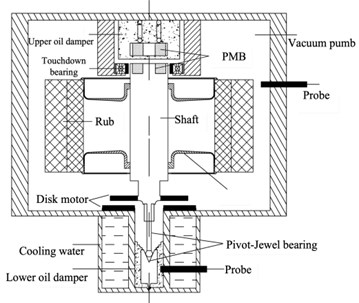A vacuum pump is used to make the container have vacuum. When the flywheel fails to damage, the vacuum container has a protective effect to prevent injury to people. The flywheel is composed of rim, hub and steel shaft. The rim is made of composite, the hub consists of aluminum alloy hub, and steel shaft. The aluminum alloy hub connects the rim to the shaft. The flywheel rotor is assembled with the disc type motor, which will drive the flywheel to a high rotating speed to store energy, and release electric energy when needed. The disk type motor has small weight and volume. Its armature winding inside the stator is cooled by recycled water, which takes away the heat of the oil damper at the same time.

The flywheel rotor is levitated by a permanent magnet bearing (PMB) at the top. The PMB includes stationary permanent magnetic ring and steel ring installed at the top of flywheel rotor.

The PMB produces axial magnetic force to reduce the load of flywheel rotor on the jewel bearing. The PMB produces small radial stiffness by closed magnetic-flux design. The permanent magnetic ring is hung in a oil-filled damper. It vibrates laterally slightly like a pendulum and is damped by viscous damping. The upper oil damper is used to suppress vibration transmitted from flywheel by magnetic field coupling. The PMB unloads 60-70 percent of the flywheel weight.

The composite flywheel rotating at high speed bears large centrifugal force, which causes resin and fiber minor damages easily. A large sudden imbalance produces huge impact force. It is difficult for the PMB to provide large enough radial stiffness to resist the impact force. The rubbing protection device is designed for this reason. The rubbing protection device is composed of rubber layer and touchdown bearing. The rubber layer is used to reduce the friction damage when the rubbing occurs. The touchdown bearing is used to support flywheel top to keep on rotating.

The pivot-jewel bearing and oil damper constitute a single point flexible support. The pivot-jewel bearing is installed at the top of damping body inside the oil damper. The oil damper is filled with vacuum pump oil. The jewel bearing is immersed in oil and lubricated. The pivot tip is spherical surface, which contacts with concave of the jewel bearing. The contact area is less than 2 mm2. The friction loss of jewel bearing is very small. The oil damper produces damping through dynamic viscosity of the oil, which is unlike squeeze oil film damper. There is no oil supply for the oil damper, which is unlike general sliding bearing.

Fig. 2 shows a dynamic model of the flywheel shafting system. In order to obtain a simple model, the vibration displacements in the $x$ and $y$ directions are expressed as complex vectors $r$ ($r=x+iy$). The meaning of the symbols in the Fig. 2 is shown in the Nomenclature.

The following simplifications are made in the modeling. 1) Oil in the upper and lower damper provides linear viscous damping. 2) The hysteresis loss of PMB is very little. 3) The flywheel rotor is considered as a rigid body. 4) The eddy current loss of the disc motor is very small and not considered. 5) The magnetic pull force of the disc motor is very small and not considered. 6) The lateral vibrations are small and can be considered as linear.7) The pivot is small and soft, its stiffness is much lower than that of flywheel. 8) The upper and lower oil dampers have linear stiffness and damping coefficient.

Fig. 2Dynamic model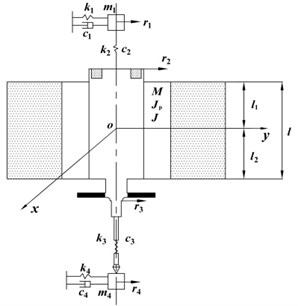### 2.2. Damping and stiffness coefficient measurement

The upper and lower oil dampers are very important for the flywheel system. As shown in Fig. 1, the dampers work like a pendulum with a single degree of freedom. The damper has three dynamic parameters. Equivalent mass of upper oil damper is provided by the no-rotating part of the PMB. The equivalent stiffness is ensured by a cantilever beam in the upper oil damper. The dynamic viscosity of the damping oil ensures equivalent damping coefficient.

The damping body provides the equivalent mass of the lower oil damper. Three small stiffness springs ensure the equivalent stiffness. The dynamic viscosity of damping oil determines equivalent damping coefficient.

Based on vibration theory of the single degree of freedom, the stiffness and damping coefficients are obtained:

1
$k=m{\omega }_{n}^{2},$
2
$c=2m\zeta {\omega }_{n}.$

In Eq. (1), $\zeta$ is the damping ratio, ${\omega }_{n}$ is the natural angular frequency in Eq. (2). A damped single degree of freedom system can be described by Eq. (3):

3
$x=A{e}^{-\sigma t}\mathrm{s}\mathrm{i}\mathrm{n}\left({\omega }_{d}t+\alpha \right).$

The vibration waveform is shown in Fig. 3. The vibration amplitude is attenuated by following the exponential decay. The attenuation coefficient is $\sigma$. The amplitude ratio is $\eta$:

4
$\eta =\frac{{X}_{i}}{{X}_{i+1}}=\frac{{X}_{i+1}}{{X}_{i+2}}=\dots ={e}^{\sigma {T}_{d}}.$

Fig. 3Vibration waveform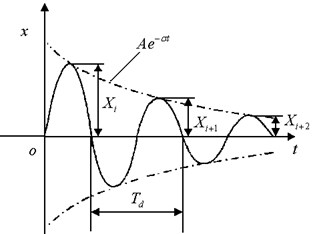The larger $\sigma$ and $\eta$ have more faster decay rate. The natural logarithm of $\eta$ is logarithm amplitude ratio $\delta$:

5
$\delta =\frac{1}{n}\mathrm{l}\mathrm{n}\frac{{X}_{i}}{{X}_{i+n}}=\sigma {T}_{d}.$

The damped natural angular frequency is ${\omega }_{d}$, and the natural damping period is ${T}_{d}$, when 01:

6
${T}_{d}=\frac{2\pi }{{\omega }_{d}}=\frac{2\pi }{{\omega }_{n}\sqrt{1-{\xi }^{2}}}=\frac{{T}_{n}}{\sqrt{1-{\xi }^{2}}},$
7
${\omega }_{n}=\frac{2\pi }{{T}_{d}\sqrt{1-{\xi }^{2}}}.$

Substituting Eq. (6) into Eq. (5), Eq. (8) is obtained:

8
$\zeta =\frac{\delta }{\sqrt{4{\pi }^{2}+{\delta }^{2}}}.$

Simultaneous Eq. (8), Eq. (7), Eq. (1), and Eq. (2), Eq. (9) is obtained:

9

The responses of the dampers were measured by the eddy current sensors under excess excitations. The vibration signal was collected by a TDK2000 oscilloscope after excitation. By exciting the damping body in the lower oil damper at different frequencies, a single degree of freedom vibration signal can be obtained. Several different types of excitation waveforms were shown in Fig. 4. The excitation waveform was shown in Fig. 4(a) when dampers are without oil. Fig. 4(b-d) showed excitation waveforms with a larger damping coefficient. The VF68 vacuum pump oil was finally selected as a damping oil, which had very good dynamic viscosity characteristics and not easy to emulsify.

It was found that the dynamic parameters of the upper and lower dampers were very little affected by the excitation frequencies, which indicated that the rotating speed of the flywheel had a little effect on the dynamic parameters of dampers. The average values of multiple measurements were preferred. For the dynamic parameters shown in Fig. 2, ${m}_{1}$ is 0.38 kg, ${k}_{1}$ is 1760 N/m, ${c}_{1}$ is 80 N·s/m, ${m}_{4}$ is 0.86 kg, ${k}_{4}$ is 3.2e4 N/m, ${c}_{1}$ is 480 N·s/m.

The stiffness ${k}_{2}$ was obtained by equivalent current method [22, 23]. The pivot was considered as a beam, and the stiffness ${k}_{3}$ was determined based on the beam theory [18, 24].

Fig. 4Excitation waveforms with different damping medium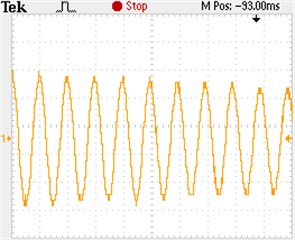a) Almost no damping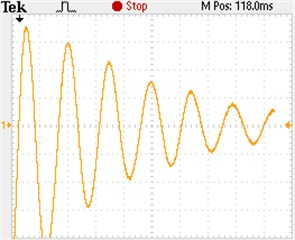b) Underdamping 1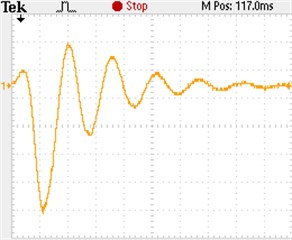c) Underdamping 3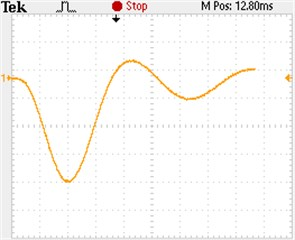d) Underdamping 4

### 2.3. Dynamic model of flywheel rotor system

Fig. 2 shows a model of flywheel rotor system. ${T}_{i}$ indicates the kinetic energy, ${U}_{i}$ is the potential energy, and ${Z}_{i}$ is the consumed energy function. The rigid body dynamics modeling was relatively reliable and effective [25, 26].

(1) Modeling of the upper oil damper:

10
$\begin{array}{l}{T}_{1}=\frac{1}{2}{m}_{1}\left({\stackrel{˙}{x}}_{1}^{2}+{\stackrel{˙}{y}}_{1}^{2}\right),\\ {U}_{1}=\frac{1}{2}{k}_{1}\left({x}_{1}^{2}+{y}_{1}^{2}\right),\\ {Z}_{1}=\frac{1}{2}{c}_{1}\left({\stackrel{˙}{x}}_{1}^{2}+{\stackrel{˙}{y}}_{1}^{2}\right).\end{array}$

(2) Modeling of the PMB:

11
${U}_{2}=\frac{1}{2}{k}_{2}\left({\left({x}_{2}-{x}_{1}\right)}^{2}+{\left({y}_{2}-{y}_{1}\right)}^{2}\right),$
${Z}_{12}=\frac{1}{2}{c}_{2}\left\{{\left[\left({\stackrel{˙}{x}}_{2}-{\stackrel{˙}{x}}_{1}\right)+\omega \left({y}_{2}-{y}_{1}\right)\right]}^{2}+{\left[\left({\stackrel{˙}{y}}_{2}-{\stackrel{˙}{y}}_{1}\right)-\omega \left({x}_{2}-{x}_{1}\right)\right]}^{2}\right\}.$

(3) Modeling of the flywheel:

12

(4) The pivot:

13
${U}_{3}=\frac{1}{2}{k}_{3}\left({\left({x}_{4}-{x}_{3}\right)}^{2}+{\left({y}_{4}-{y}_{3}\right)}^{2}\right),$
${Z}_{34}=\frac{1}{2}{c}_{3}{\left[\left({\stackrel{˙}{x}}_{3}-{\stackrel{˙}{x}}_{4}\right)+\omega \left({y}_{3}-{y}_{4}\right)\right]}^{2}+{\left[\left({\stackrel{˙}{y}}_{3}-{\stackrel{˙}{y}}_{4}\right)-\omega \left({x}_{3}-{x}_{4}\right)\right]}^{2}.$

(5) The lower oil damper:

14
${T}_{3}=\frac{1}{2}{m}_{4}\left({\stackrel{˙}{x}}_{4}^{2}+{\stackrel{˙}{y}}_{4}^{2}\right),$
${U}_{3}=\frac{1}{2}{k}_{4}\left({x}_{4}^{2}+{y}_{4}^{2}\right),$
${Z}_{3}=\frac{1}{2}{c}_{4}\left({\stackrel{˙}{x}}_{4}^{2}+{\stackrel{˙}{y}}_{4}^{2}\right).$

The dynamic equation of the flywheel rotor system is obtained by Lagrange Eq. (15):

15
$\frac{d}{dt}\left(\frac{\partial T}{\partial {\stackrel{˙}{q}}_{i}}\right)-\frac{\partial T}{\partial {q}_{i}}+\frac{\partial U}{\partial {q}_{i}}+\frac{\partial Z}{\partial {q}_{i}}={\stackrel{-}{Q}}_{i},$
16
$\left[M\right]\left\{\stackrel{¨}{r}\right\}+\left(\left[C\right]-i\omega \left[H\right]\right)\left\{\stackrel{˙}{r}\right\}+\left(\left[K\right]-i\omega \left[{C}_{i}\right]\right)\left\{r\right\}=\left\{0\right\}.$

The matrix form of Eq. (16) is as follows:

Eq. (16) is solved by using the state vector method, its whose solution form is:

17

The amplitude and frequency of the complex form are expressed as follows:

18

$s=\lambda +i\omega .$

In Eq. (18), $\lambda$ is modal decay index, $\omega =2\pi f$ is the modal angular frequency, and $f$ is the modal frequency. $\zeta =-\lambda /\omega$ is the modal damping ratio. The dynamic of flywheel system is stable if $\lambda$ is positive. The forced vibration equation is obtained by Lagrange Eq. (15) if the residual unbalance is $\left\{f\right\}=\left\{u\right\}{\omega }^{2}{e}^{i\omega t}$ at the flywheel rotor top and bottom.

The steady state unbalance response was calculated from Eq. (19):

19
$\left[M\right]\left\{\stackrel{¨}{r}\right\}+\left(\left[C\right]-i\omega \left[H\right]\right)\left\{\stackrel{˙}{r}\right\}+\left[K\right]\left\{r\right\}=\left\{u\right\}{\omega }^{2}{e}^{i\omega t}.$

## 3. Dynamic simulation of flywheel rotor system

The dynamic parameters of the flywheel rotor bearing system were as shown in Table 1.

As described in Section 2.2, the dynamic parameters of dampers were determined by the free vibration attenuation method. The other parameters were obtained by calculation and measurements.

Table 1Dynamic parameters of flywheel shafting

 Parameters Upper damper PMB Flywheel Pivot Lower damper $\mathrm{m}$ /kg 0.72 – 7.5 – 0.86 ${\mathrm{J}}_{\mathrm{d}}$ /kg·m2 – – 4.2e-2 – – ${\mathrm{J}}_{\mathrm{p}}$ /kg·m2 – – 5.6e-2 – – $\mathrm{K}$ / N·m-1 800 2200 – 6.8e4 7.2e4 / N·s·m-1 500 10 – 10 370 $\mathrm{H}$ / cm – – 13 – –

### 3.1. Mode shapes

The flywheel shafting had four pairs of main vibration modes. Only the forward modes were calculated and analyzed below. The modal frequencies and mode shapes of the shafting when rotation frequency is 0 Hz as shown in Fig. 5. The four points from top to down represent the upper damper, the top of flywheel rotor, the bottom of flywheel rotor, and the lower oil damper. The vector of each point represents the vibration amplitude and phase.

Fig. 5Modal frequency and mode shape (0 Hz)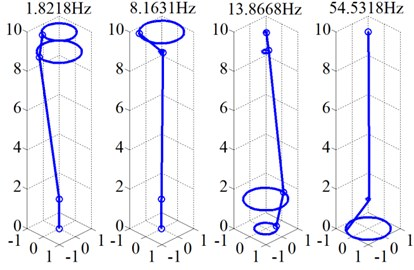The first four modal frequencies are 1.82 Hz, 8.16 Hz, 13.87 Hz and 54.53 Hz, corresponding to cylindrical mode, upper damper mode, conical mode and lower damper mode. The vibration amplitude of the upper damper is much bigger in the upper damper mode, which is displayed as circular motion of damping body, while flywheel is fixed. The vibration amplitude of the lower damper is much bigger in the lower damper mode, which is displayed as circular motion of damping body, while the flywheel is fixed.

The exchanges of mode shapes have appeared when the flywheel speed reaches 660 rpm-720 rpm. As shown in Fig. 6 and Fig. 7, the upper damper mode exchanges with the cylindrical mode. The main reason is that their modal frequencies are very close, which is due to the stiffness of both upper oil damper, and the PMB is very small. This is very different from the conventional support technology.

Fig. 6Modal frequency and mode shape (11 Hz)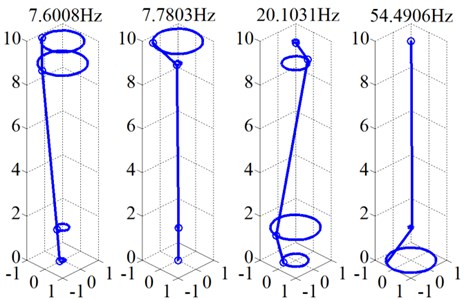Fig. 7Modal frequency and mode shape (12 Hz)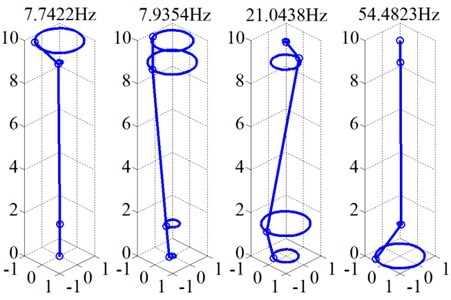As the same principle with the upper support, the frequencies of the conical mode and the lower damper mode are very close because of their stiffness almost the same and very small. It can be seen from Fig. 8 and Fig. 9, both modes exchanges with each other when the flywheel rotates at 2280 rpm-2340 rpm.

Fig. 8Modal frequency and mode shape (38 Hz)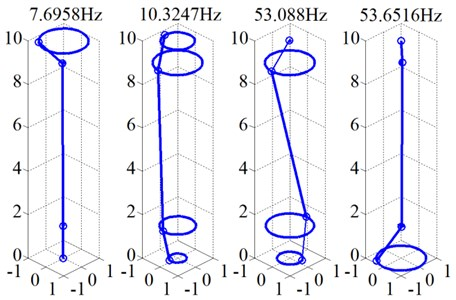As the rotating speed increases, four modal frequencies are changing. For example, Fig. 5 and Fig. 10 shows the cylindrical mode frequency increases from 1.82 Hz to 11.02 Hz as the speed rises to 21000 rpm, and the conical mode frequency increases from 13.87 Hz to 466.99 Hz. The modal frequencies of both dampers are basically unchanged.

Fig. 9Modal frequency and mode shape (39 Hz)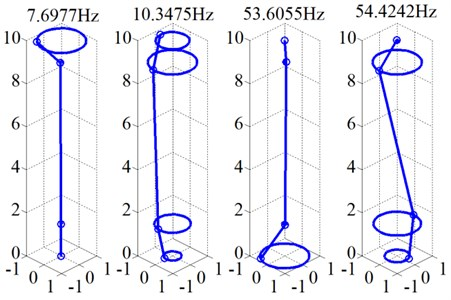Fig. 10Modal frequency and mode shape (350 Hz)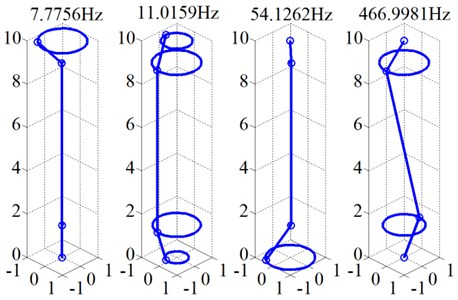### 3.2. Critical speeds

Fig. 11 shows the Campbell diagram. ‘UDF’ and ‘UDB’ indicate the mode of upper damper. ‘1F’ and ‘1B’ indicate the mode of the first-order mode. ‘2F’ and ‘2B’ indicate the second-order mode respectively. ‘LDF’ and ‘LDB’ indicate the mode of lower damper respectively.

When the flywheel is stationary, the modal frequencies of the forward and backward precessions for each degree of freedom are equal and appear in pairs. The direction of the gyroscopic vector is the same as the elastic force when the forward precession happens, that is equal to increase the stiffness of the flywheel shafting. As the speed increases, the forward modal frequencies become bigger, and the backward modal frequencies become smaller.

When the cylindrical mode frequency is close to and exceeds the upper damper mode frequency, the two modes will have a mode shape exchange; the frequency range is about 11 to 12 Hz. Similarly, when the conical mode frequency is close to the lower damper mode frequency, a mode shape exchange will also take place; the frequency range is about 38 to 39 Hz. The critical speeds of the flywheel rotor system are 450 rpm and 3300 rpm. The corresponding mode shapes are the cylindrical mode and the lower damper mode.

### 3.3. Modal damping ratios

The stability indices of the flywheel system are the modal damping ratios. By solving Eq. (17), the modal damping ratios are obtained. Fig.12 shows that it changes with the rotating speed. The backward precession is generally considered stable; the forward precession is concerned only here. For the forward precession mode, the dynamics of flywheel system is stable when the modal damping ratio is positive. The greater the modal damping ratio is, the better the stability is.

Fig. 11Campbell diagram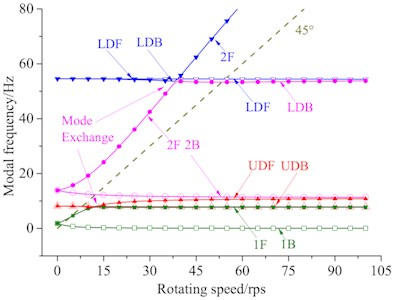Fig. 12Modal damping ratios of flywheel rotor system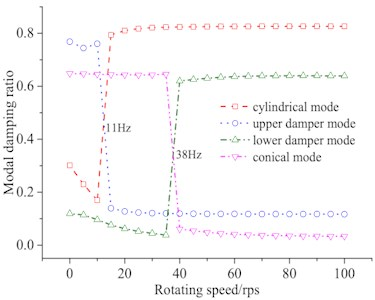Fig. 12 shows the four types of mode are stable. The damping ratio of the cylindrical mode suddenly increases from 0.13 to 0.8, while the damping ratio of the upper damper mode suddenly drops from 0.78 to 0.10. The corresponding frequency at turning point is 11 Hz that is the mode shape exchange frequency. Similarly, the damping ratio of the lower damper mode suddenly increases from 0.06 to 0.63, while the damping ratio of the conical mode suddenly drops from 0.65 to 0.18. The corresponding frequency at the turning point is 38 Hz, that is the mode shape exchange frequency. It is obvious the mode damping ratio of shafting is interrupted and discontinuous due to the mode shape exchange. This is very different from the ordinary rotor system.

### 3.4. Dynamic influences of stiffness in flywheel shafting

Fig. 13 shows the relationship between the upper damper stiffness and the modes. The cylindrical and conical mode frequencies are mainly focused on it. With increasing stiffness ${k}_{1}$, the frequencies of the conical mode almost do no change at all; that indicates the stiffness ${k}_{1}$ has a negligible effect on the conical mode. And the cylindrical mode frequencies increase greatly within the range of 7 Hz to 14 Hz. The stiffness ${k}_{1}$ has a big effect on the cylindrical mode frequencies.

The modal damping ratio is also a very important dynamic parameter. The modes with small modal damping ratios threaten the flywheel life. The larger modal damping ratio, which accelerates decaying the natural whirl, is preferred. As shown in Fig. 13, the stiffness ${k}_{1}$ has a little effect on the modal damping ratio of the lower damper and the upper damper. However, with increasing stiffness ${k}_{1}$, the cylindrical mode damping ratio decreases, and when the stiffness is larger than 2500 N/m, the modal damping ratio drops sharply from 0.6 to 0.1. On the contrary, with increasing stiffness ${k}_{1}$, the conical modal damping ratios essentially unchanged, and when the stiffness is larger than 2500 N/m, the modal damping ratios have a sharp increase from 0.1 to 0.6. Therefore, larger stiffness reduces the stability of the cylindrical mode but improves the stability of the conical mode.

Fig. 14 shows the PMB stiffness influence on mode frequencies and modal damping ratios. With increasing stiffness ${k}_{2}$, the frequencies of the conical mode are basically unchanged. When ${k}_{2}$ is greater than 3800 N/m, the mode frequencies have a large increase. And the frequencies of the cylindrical mode increase greatly within the range from 6.8 Hz to 11 Hz. The stiffness ${k}_{2}$ has a big effect on the cylindrical mode frequencies. However, when ${k}_{2}$ is greater than 3800 N/m, the mode frequencies decrease lightly. The point at which ${k}_{2}$ is equal to 3800 N/m is an inflection point.

Fig. 13Upper damper stiffness influence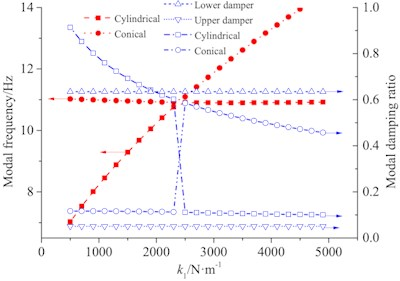Fig. 14PMB stiffness influence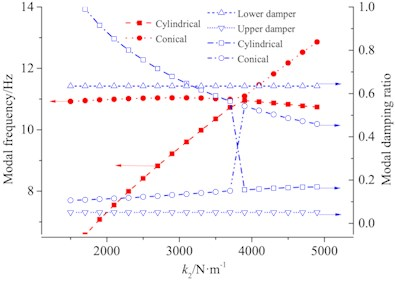The PMB stiffness ${k}_{2}$ has a little effect on the modal damping ratio of the lower damper and the upper damper. However, with increasing ${k}_{2}$, the cylindrical modal damping ratios decrease, and when ${k}_{2}$ is larger than 3800 N/m, the modal damping ratios drop sharply from 0.55 to 0.15. On the contrary, with increasing ${k}_{2}$, the conical modal damping ratios are essentially unchanged, and when ${k}_{2}$ is larger than 3800 N/m, the modal damping ratios have a sharp increase from 0.15 to 0.55. The larger stiffness ${k}_{2}$ reduces the stability of the cylindrical mode, but increases the stability of the conical mode. This rule is consistent with what ${k}_{1}$ shows.

Fig. 15 shows the pivot stiffness influence on mode frequencies and modal damping ratios. With increasing stiffness ${k}_{3}$, the frequencies of the cylindrical mode are basically unchanged. And the frequencies of the conical mode increase slowly within the range from 10 Hz to 13.5 Hz. The stiffness ${k}_{3}$ has a big effect on the conical mode frequencies.

The cylindrical mode and the upper damper mode are almost unaffected by the pivot stiffness ${k}_{3}$. However, with increasing ${k}_{3}$, the lower damper damping ratios decrease from 0.7 to 0.3. On the contrary, with increasing ${k}_{3}$, the conical modal damping ratios have a slight increase from 0.15 to 0.2. It can be said larger stiffness ${k}_{3}$ improves the conical mode stability, however, it reduces the lower damper mode stability.

Fig. 15Pivot stiffness influence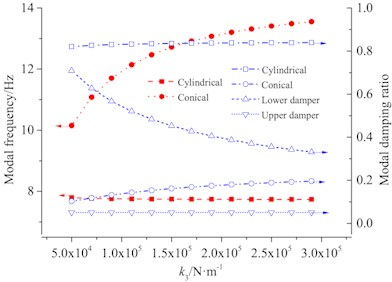Fig. 16Lower damper stiffness influence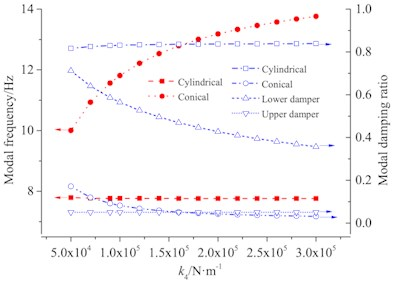As shown in Fig. 16, with increasing stiffness ${k}_{4}$, the frequencies of the cylindrical mode are basically unchanged. And the frequencies of the conical mode increase slowly within the range from 10 Hz to 13.5 Hz. The stiffness ${k}_{4}$ has a big effect on the conical mode frequencies. This rule is consistent with what ${k}_{3}$ shows.

The ${k}_{4}$ has a little effect on the modal damping ratio of the first three modes. However, with the increase of ${k}_{4}$, the lower damper damping ratios decrease from 0.7 to 0.35. So, the larger stiffness ${k}_{4}$ reduces the lower damper mode stability. This rule is consistent with what ${k}_{3}$ shows.

### 3.5. Dynamic influences of damping in flywheel shafting

The damping of PMB and pivot is negligible. So, it is worth focusing on mainly the damping of the upper and lower dampers. Fig. 17 shows that, with increasing damping ${c}_{1}$, the frequencies of the conical mode are basically unchanged. And the frequencies of the cylindrical mode decrease slowly within the range from 10 Hz to 6.5 Hz. The damping ${c}_{1}$ has a big effect on the cylindrical mode frequencies.

Fig. 17 shows that, with increasing ${c}_{1}$, the cylindrical mode damping ratios increase from 0.1 to 1.0. Therefore, the larger damping makes the stability of the upper damper mode be better. However, ${c}_{1}$ hardly affect the conical mode, the lower and upper damper mode.

As shown in Fig. 18, with increasing the damping ${c}_{4}$, the frequencies of the cylindrical and conical mode are basically unchanged. The damping ${c}_{4}$ has little effect on the cylindrical, conical and upper damper modes. However, with increasing ${c}_{4}$, the damping ratios increase from 0.1 to 1.0. Therefore, the larger damping ${c}_{4}$ makes the stability of the lower damper mode be better.

Fig. 17Upper damper damping influence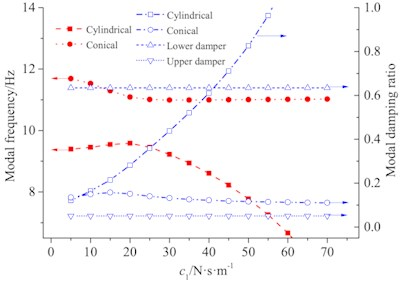Fig. 18Lower damper damping influence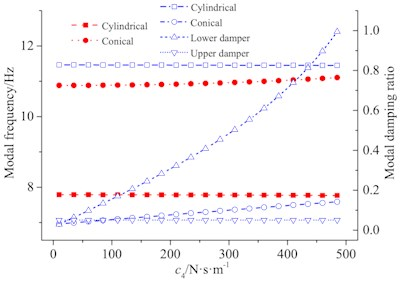## 4. Experimental verification

### 4.1. Critical speeds and forced vibration test

The following three parts compose of the FESS experimental set-up: flywheel rotor-bearing system, sensors, data collector and analyzer.

The flywheel rotor-bearing system is described in detail in Section 2.1. The sensor system mainly consists of photoelectric speed sensors and eddy current displacement sensors. For the eddy current displacement sensors, its supply voltage is ±15 V, probe diameter is 5 mm, gap-voltage is –10-10 V, and the sensitivity of eddy current displacement sensors is 20 mV/μm. Two probes are located at the flywheel top to measure the vibration displacement of $x$ and $y$ directions respectively. Two probes are installed in the lower damper to collect the vibration displacement of damping body in $x$ and $y$ directions respectively. And the NI DAQ and a computer were used to collect and analyze the vibration data. Its maximum sampling frequency is 100 kHz, A/D accuracy is 24 bit, and channel number of NI DAQ is 16.

The rotating speed of the rotor is obtained by a photoelectric sensor. The test data are inputted to the computer, and analyzed by the NI DAQ. The vibration amplitude is measured by eddy current displacement sensors, and the time domain signals are converted to frequency domain signals through the SFFT algorithm.

The residual unbalance of upper and lower ends of the flywheel rotor were 5.1g·cm$\angle$275°and 3.2g·cm$\angle$332°respectively. The forced vibration response of the flywheel can be calculated by Eq. (19). And the calculated data are further compared with the experimental data.

In the calculation of Section 2.2, it was known that the critical speeds of the flywheel shafting were 450 rpm (cylindrical mode) and 3300 rpm (lower damper mode).

The forced vibration of the flywheel rotor was measured by an eddy current damper installed in the upper end of the flywheel and the lower oil damper. Fig. 19 shows the vibration response of the upper end of the flywheel. The critical speed measured by the test was 370 rpm, its corresponding theoretical calculation was 450 rpm. After passing the critical speeds, the flywheel ran smoothly to 36000 rpm. The peak value at critical speed was approximately 30 μm.

Fig. 20 shows the vibration amplitude of the lower oil damper. The measured value of the critical speed was 3500 rpm, and the corresponding calculated value was 3300 rpm. The error between these two was small in the critical speed region, corresponding to a vibration peak of 10 μm. The peak amplitude was about 8 μm in the first test, that was slightly smaller than the numerical result. In the second test, the vibration peak was almost identical to the theoretical calculation. As the speed increased, the dynamic stability of the lower damper was good, and the damping effect was prominent.

After using the PMB and a pendulum oil damper as its upper support, the vibration amplitude of the upper end of the flywheel is decreased, and the amplitude of the lower end of the flywheel is also the same. However, the influence of PMB on the 1st critical speed is little, that is mainly due to the radial stiffness of the PMB is very small. The pendulum oil damper has better effect of suppressing amplitude of the 1st critical speed.

Fig. 19Amplitude of upper end of flywheel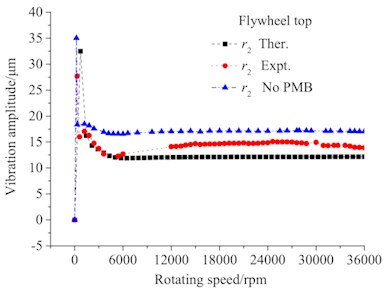Fig. 20Vibration amplitude of lower oil damper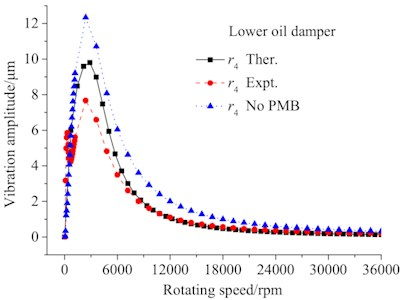### 4.2. Damping and stiffness effect

For the lower damper shown in Fig. 1, its stiffness and damping parameters are easily adjusted. In section 1.2, this picture was demonstrated more clearly.

The damping coefficient ${c}_{4}$ can be adjusted by the type of damping oil, e.g., by mixing two damping oils, Mobil 1 and Mobil 68. The stiffness ${k}_{4}$ changes with the help of springs having different thickness. The spin test was performed using two different sets of stiffness and damping coefficients. The first set of coefficients, ${k}_{4}$ was 8.7e4 N/m and ${c}_{1}$ was 460 N·s/m. The second set of coefficients, ${k}_{4}$ was 5.6e4 N/m, and ${c}_{1}$ was 510 N·s/m.

As shown in Fig. 16, with increasing ${k}_{4}$, the conical mode frequencies increase slowly, the larger ${k}_{4}$ makes the stability of the lower damper mode be worse. As shown in Fig. 20, with increasing ${c}_{4}$, the larger damping makes the stability of the lower damper mode be better. The experimental data was used to verify the theoretical results above.

The flywheel top vibration with different parameters was shown in Fig. 21. When ${k}_{4}$ was 8.7e4 N/m, its corresponding critical speed was bigger than numerical solution, and when ${k}_{4}$ was 5.6e4 N/m, its corresponding critical speed was smaller. The bigger ${k}_{4}$ has bigger critical speed. The damping effect showed different rules. In the critical speed region, the larger the damping was, the larger the amplitude was. After passing the critical speed, a larger damping leads to a smaller amplitude. This was a phenomenon that traditional bearings did not have.

Fig. 21Flywheel top vibration with different parameters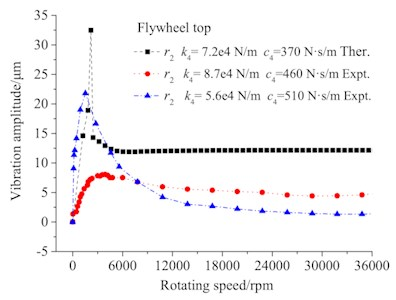Fig. 22Lower damper vibration with different parameters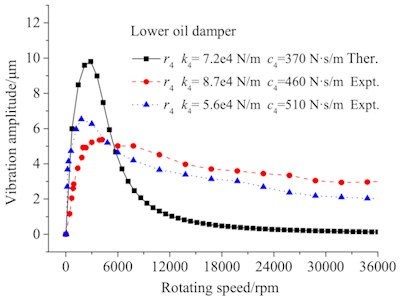It was observed that vibration of the lower damper had different characteristics. Fig. 22 showed the lower damper vibration with different parameters. It can be seen that the greater stiffness had the bigger modal frequency. As the stiffness increased, the critical speed increased. However, the effect of damping on vibration was different from the effect of stiffness on vibration. In the critical speed region, the test value was smaller than the calculated value, so the larger damping led to the larger amplitude, and the damping did not show the effect of vibration suppression. After passing the critical speed, the test value was greater than the calculated value, but the larger damping had the smaller amplitude. It may be a larger ${k}_{4}$ value which makes the stability of the lower damper mode be worse. It can be explained by the fact that the lower damper absorbed the vibrational energy of the rotor, causing its own vibration to increase. And the bigger damping has better vibration absorption effect.

## 5. Conclusions

1) The lower support system was composed of a pivot-jewel bearing and a spring oil damper, and a permanent magnetic bearing and a simple pendulum oil damper were utilized as its upper support. This type of hybrid support had very little friction loss, simple and long lifetime without maintenance. There is no need for complex and costly control systems.

2) The dynamic equation of the flywheel rotor system is established based on the Lagrange equation. The upper and lower bearing parameters were measured by the excitation with a single degree of freedom. By solving the dynamic equation, the mode shapes, Campbell diagram, critical speeds, and modal damping ratios were obtained. It is obvious the mode damping ratio of shafting is interrupted and discontinuous due to the mode shape exchange. This is very different from the ordinary rotor system. The flywheel rotor system has two critical speeds, 450 rpm, and 3300 rpm. The corresponding mode shapes are the cylindrical mode and the lower damper mode.

3) The consistency between the dynamic model simulation and the experimental results is very good, indicating that the theoretical model is correct. After passing the critical speed, the amplitude drops sharply. This is a self-centering effect. As the speed increases, the dynamic stability of the lower damper was good, and the damping effect was prominent. It may be the larger ${k}_{4}$ value which makes the stability of the lower damper mode be worse. It can be explained by the fact that the lower damper absorbed the vibrational energy of the rotor, causing its own vibration to increase. And the bigger damping has better vibration absorption effect.

#### Acknowledgements

This work was supported by the Science and Technology Program of the Beijing Municipal Education Commission (Grant Number: KM201911232007) and the Supplementary and Supportive Project for Teachers at Beijing Information Science and Technology University (2018-2020) (Grant Number: 5029011103) and Promote the Connotation Development of Universities-Key Research and Cultivation Projects (Grant Number: 5211910938) and the National Natural Science Foundation of China (Grant Number: 11402267).